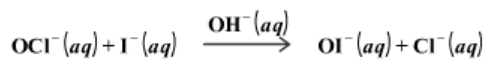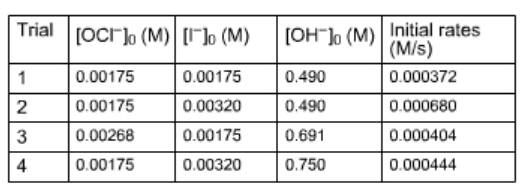# Problem: Consider this initial-rate data at a certain temperature for the reaction described byDetermine the rate law and the value of the rate constant for this reaction.

###### FREE Expert Solution

We’re being asked to calculate the value of the rate constant (k) based on the reaction and data given.

Recall that the rate law only focuses on the reactant concentrations and has a general form of:

k = rate constant
A & B = reactants
x & y = reactant orders

We’re going to calculate the rate law and the rate constant using the following steps:

91% (310 ratings)###### Problem Details

Consider this initial-rate data at a certain temperature for the reaction described byDetermine the rate law and the value of the rate constant for this reaction.

Frequently Asked Questions

What scientific concept do you need to know in order to solve this problem?

Our tutors have indicated that to solve this problem you will need to apply the Rate Law concept. You can view video lessons to learn Rate Law. Or if you need more Rate Law practice, you can also practice Rate Law practice problems.